# Mathematical Expansion of the Dipole Moment

### Hellman-Feynman Theorem

The Hellman-Feynman Theorem, which expresses the dipole moment as minus derivative of the energy of the system with respect to the field. This equation expresses the response of a molecule which is 2nd order in terms of the energy of the molecule and first order in terms of the dipole moment of the molecule (1st order or 2nd order with respect to the field).$\overrightarrow {\mu} = - \frac {\delta E} {\delta \overrightarrow{F}}\,\!$$\overrightarrow{\mu} = \overrightarrow{\mu^\circ} + \alpha \overrightarrow{F} + \frac {1} {2!} \beta \overrightarrow{F}\overrightarrow{F} + \frac {1} {3!} \gamma \overrightarrow{F}\overrightarrow{F}\overrightarrow{F}\,\!$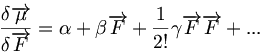$\frac {\delta \overrightarrow{\mu}}{\delta \overrightarrow{F}} = \alpha + \beta \overrightarrow{F} + \frac {1} {2!} \gamma \overrightarrow{F}\overrightarrow{F} + ...\,\!$

### Stark Energy Expression

Alpha is the linear polarizability or the first order polarizability. It describes how the molecule responds in terms of the modification of its ground state energy or of its dipole moment, in the presence of the field at the limit where the field goes to 0. Thus, α can be cast either as the 1st order derivative of the dipole moment with respect to the field when the field tends to 0 or minus the 2nd order derivative of the ground state energy of the molecule with respect to the field when the field goes to 0.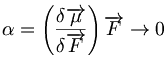$\alpha = \left( \frac {\delta\overrightarrow{\mu}}{\delta \overrightarrow{F}}\right) \overrightarrow{F} \rightarrow 0 \,\!$$= - \left( \frac {\delta^2 E}{\delta \overrightarrow{F}^2}\right) \overrightarrow{F} \rightarrow 0 \,\!$

Beta is the 2nd order derivative of the dipole moment with respect to the field when the field goes to 0. Beta will be referred to as the 2nd order polarizability of the molecule. This can also be derived from the stark energy expression which is the 3rd order derivative of the energy with respect to the field when the field tends to 0.$\beta = \left( \frac {\delta^2 \overrightarrow{\mu}}{\delta \overrightarrow{F}^2}\right) \overrightarrow{F} \rightarrow 0 \,\!$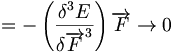$= - \left( \frac {\delta^3 E}{\delta \overrightarrow{F}^3}\right) \overrightarrow{F} \rightarrow 0 \,\!$

Lastly, the γ term corresponds to the 3rd order derivative of the dipole moment or minus the 4th order derivative of the energy with respect to the field at the limit where the field goes to 0. γ will be referred to as the 3rd order polarizability of the molecule.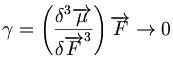$\gamma = \left( \frac {\delta^3 \overrightarrow{\mu}}{\delta \overrightarrow{F}^3}\right) \overrightarrow{F} \rightarrow 0 \,\!$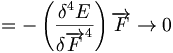$= - \left( \frac {\delta^4 E}{\delta \overrightarrow{F}^4}\right) \overrightarrow{F} \rightarrow 0 \,\!$

Stark energy describes the evolution of the energy of a system of particles in the presence of an electric field F. In the Stark energy expression, γ corresponds to a 4th order term. However the in common terminology α is referred to as the linear polarizability, β the 2nd order polarizability, and γ the 3rd order polarizability. The Hellman-Feynman Theorem is the origin of these terms. Here it is presented as a Taylor series expansion, sometimes one uses a power series expansion.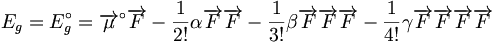$E_g = E^\circ_g = \overrightarrow{\mu}^\circ \overrightarrow{F} - \frac {1} {2!} \alpha \overrightarrow{F}\overrightarrow{F} - \frac {1} {3!} \beta \overrightarrow{F}\overrightarrow{F}\overrightarrow{F} - \frac {1} {4!} \gamma \overrightarrow{F}\overrightarrow{F}\overrightarrow{F}\overrightarrow{F}\,\!$$= E^\circ_g - \overrightarrow{\mu}\overrightarrow{F}\,\!$

### Electric dipole approximation

The stark energy expression states that the ground state energy of the molecule in the presence of the field is the ground state energy in the absence of the field minus μF. Thus, μF is considered the interaction between the field and the molecule. The electric dipole approximation is that only the electric field component of light influences dipole moment. The magnetic component is ignored. In most instances in the literature, this approximation is assumed but not stated. The expression for the dipole moment is expressed as :$\mu^\circ + \alpha F + \beta F^2 ... \,\!$

and so on, without paying any attention to where that expression comes from.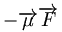$- \overrightarrow{\mu}\overrightarrow{F}\,\!$

in dimensional analysis: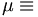$\mu \equiv\,\!$ charge * distance$F \equiv\,\!$ volt/distance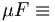$\mu F \equiv\,\!$ charge * volt :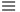$\equiv\,\!$ energy

After the electric dipole, the next two terms are the electric quadrupole and the magnetic dipole. 99% of the time only the electric dipole is considered. This represents the difference in energy between the perturbed state and the unperturbed state of the molecules. Thus, this must have an energy dimension. μ is the dipole moment which is the charge times the distance, and the electric field is volt over distance. When you multiply these terms, the expression has the dimension charge times volt or ev, and ev is an energy unit. This will be used in perturbation theory.

The stark energy expression states that the ground state energy of the molecule in the presence of the field is the ground state energy in the absence of the field minus μF. Thus, μF is considered the interaction between the field and the molecule. The electric dipole approximation is that only the electric field component of light influences dipole moment. The magnetic component is ignored. In most instances in the literature, this approximation is assumed but not stated. The expression for the dipole moment is expressed as$\mu^\circ + \alpha F + \beta F^2...\,\!$

and so on, without paying any attention to where that expression comes from.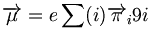$\overrightarrow{\mu} = e \sum(i) \overrightarrow{\pi}_i 9i\,\!$

After the electric dipole, the next two terms are the electric quadrupole and the magnetic dipole. 99% of the time only the electric dipole is considered. This represents the difference in energy between the perturbed state and the unperturbed state of the molecules. Thus, this must have an energy dimension. μ is the dipole moment which is the charge times the distance, and the electric field is volt over distance. When you multiply these terms, the expression has the dimension charge times volt or ev, and ev is an energy unit. This will be used in perturbation theory.